# SAT Math Multiple Choice Question 979: Answer and Explanation

### Test Information

Question: 979

15. If arc AB has a length of 12π and represents three-fourths of the circumference of circle O (not shown), what is the shortest distance between the endpoints of the arc?

• A. 4
• B.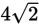• C. 8
• D.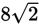Explanation:

D

Difficulty: Hard

Category: Additional Topics in Math / Geometry

Strategic Advice: Draw a sketch to visualize the situation and to reveal helpful hidden shapes.

Getting to the Answer: You're told that arc AB measures 12π and represents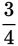of a full circumference, which means it is the major arc and sits across from an internal angle that has a measure of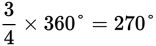. This, in turn, means minor arc AB is across from a 90° (right) angle, as shown here: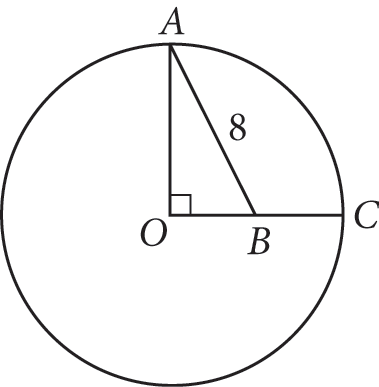Becauseof the full circumference is 12π, the full circumference itself must be 16π. A circle's circumference is equal to 2πr, so the radius of the circle is 8.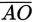and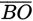are both radii and therefore equal, so ΔABO is an isosceles right (and 45-45-90) triangle with sides in the ratio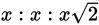. The legs (radii) have length 8, so the length of the hypotenuse (which represents the shortest distance between the endpoints of the major arc) is. (D) is therefore correct.